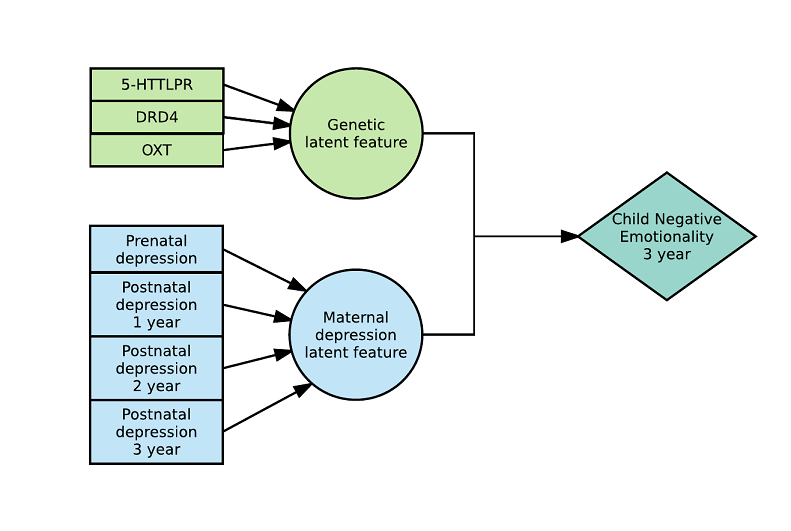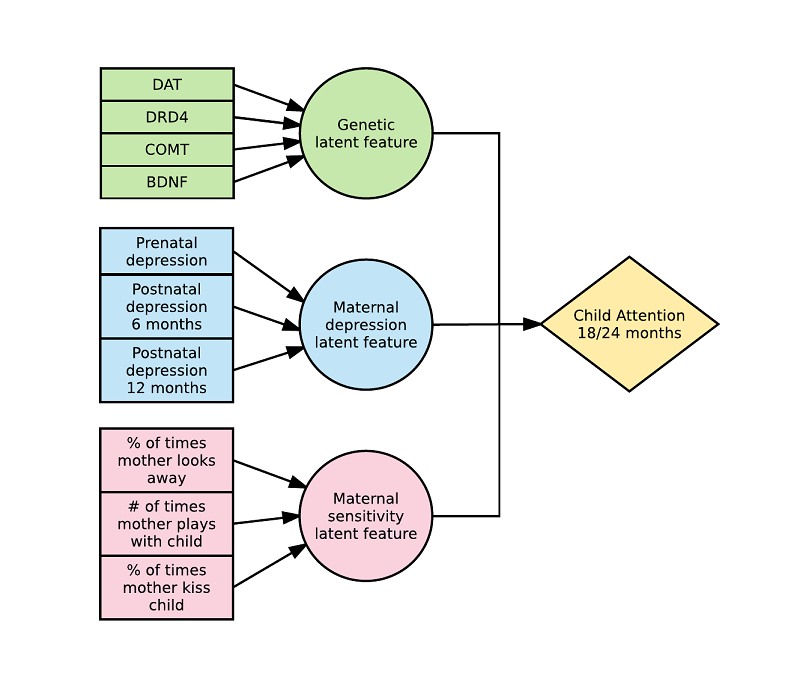# Latent Environmental and Genetic InTeraction model

The Latent Environmental and Genetic InTeraction model (LEGIT) is a model for constructing latent features inside a regression model with interactions. It all started with me wanting to be able to include multiple genes in a 3-way interaction Gene x Environment x Environment model (GxExE) rather than having to run a GxExE for each individual gene and environment. What this approach does is create latent features (let say G and E) and each feature is a weighted sum of variables (G = p1*g1 + p2*g2+ … + pk*gk and E= q1*e1 + q2*e2 + … + ql*el). Then, the latent features are interacted together however you like (Y = b0 + b1*G + b2*E + b3*G*E). All parameters inside the latent features are forced to sum to 1 (in absolute values) therefore they represent relative contributions which are very easy to interpret (ex: +20% DRD2, +40% DRD4, -40% COMT; +20% prenatal depression, +80% postnatal depression).

This is what a 2-way model with 2 latent variables looks like:This is what a 3-way model with 3 latent variables looks like:After some thoughts, I was able to come up with an easy way to train such a model using the alternating optimization algorithm. Although the model itself is non-linear, if you assume the latent features (G and E) to be known, the model is a simple linear regression. Assuming the main model parameters (b0, b1, b2, b3) and E to be known, you can expand G in terms of g1, g2, …, gk and then you also get a simple linear regression. Similarly, assuming the main model parameters (b0, b1, b2, b3) and G to be known, you can expand E in terms of e1, e2, …, ek and then you also get a simple linear regression. Therefore, one can easily estimate each model’s parts separately assuming the other parameters to be known and thus converge to the optimal solution.

What makes LEGIT really shines is that it scales linearly with the number of variables. For a 2-way GxE model with k genes and l environments, you would normally need to estimate 1 + k + s + k*s parameters but with a LEGIT model, you only need to estimate 2 + k + s parameters. Similarly, for a 3-way interaction GxE1xE2 model with k genes,  l environments in E1 and r environments in E2, you would normally need to estimate 1 + k + s + r + ks + kr + sr + krs  parameters but with a LEGIT model you only need to estimate 5 + k + s + r parameters. Because of this, LEGIT works really well with small sample sizes (n < 250).

Of course, one could use variable selection techniques (Lasso, elastic-net, etc.) to prevent having to estimate so many parameters in the first place but doing so would mean assuming that most effects are zero (which might not be true as gene effects tend to be small). This would inevitably leads to the retention of only a few strong effects while small effects or strong, but very correlated, effects would be dropped. The resulting model would likely be hard to interpret and hard to replicate in other samples. On the other hand, LEGIT is easy interpret and has a stable structure due to multiple elements contributing to each latent feature.

An R implementation for glm models is available on CRAN with a vignette showing how to use it. A SAS implementation for generalized linear mixed models is available on GitHub, it is less extensive and much slower than the R implementation but it can do mixed models.

P.S. LEGIT is a silly name, but it is indeed LEGIT!